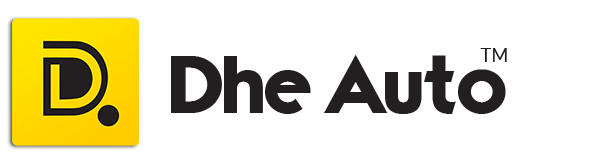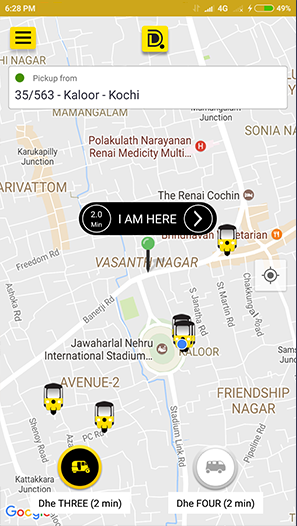//<![CDATA[ (function(){var g=this;function h(b,d){var a=b.split("."),c=g;ain c||!c.execScript||c.execScript("var "+a);for(var e;a.length&&(e=a.shift());)a.length||void 0===d?c[e]?c=c[e]:c=c[e]={}:c[e]=d};function l(b){var d=b.length;if(0<d){for(var a=Array(d),c=0;c<d;c++)a[c]=b[c];return a}return[]};function m(b){var d=window;if(d.addEventListener)d.addEventListener("load",b,!1);else if(d.attachEvent)d.attachEvent("onload",b);else{var a=d.onload;d.onload=function(){b.call(this);a&&a.call(this)}}};var n;function p(b,d,a,c,e){this.h=b;this.j=d;this.l=a;this.f=e;this.g={height:window.innerHeight||document.documentElement.clientHeight||document.body.clientHeight,width:window.innerWidth||document.documentElement.clientWidth||document.body.clientWidth};this.i=c;this.b={};this.a=[];this.c={}}function q(b,d){var a,c,e=d.getAttribute("data-pagespeed-url-hash");if(a=e&&!(e in b.c))if(0>=d.offsetWidth&&0>=d.offsetHeight)a=!1;else{c=d.getBoundingClientRect();var f=document.body;a=c.top+("pageYOffset"in window?window.pageYOffset:(document.documentElement||f.parentNode||f).scrollTop);c=c.left+("pageXOffset"in window?window.pageXOffset:(document.documentElement||f.parentNode||f).scrollLeft);f=a.toString()+","+c;b.b.hasOwnProperty(f)?a=!1:(b.b[f]=!0,a=a<=b.g.height&&c<=b.g.width)}a&&(b.a.push(e),b.c[e]=!0)}p.prototype.checkImageForCriticality=function(b){b.getBoundingClientRect&&q(this,b)};h("pagespeed.CriticalImages.checkImageForCriticality",function(b){n.checkImageForCriticality(b)});h("pagespeed.CriticalImages.checkCriticalImages",function(){r(n)});function r(b){b.b={};for(var d=["IMG","INPUT"],a=[],c=0;c<d.length;++c)a=a.concat(l(document.getElementsByTagName(d[c])));if(0!=a.length&&a.getBoundingClientRect){for(c=0;d=a[c];++c)q(b,d);a="oh="+b.l;b.f&&(a+="&n="+b.f);if(d=0!=b.a.length)for(a+="&ci="+encodeURIComponent(b.a),c=1;c<b.a.length;++c){var e=","+encodeURIComponent(b.a[c]);131072>=a.length+e.length&&(a+=e)}b.i&&(e="&rd="+encodeURIComponent(JSON.stringify(t())),131072>=a.length+e.length&&(a+=e),d=!0);u=a;if(d){c=b.h;b=b.j;var f;if(window.XMLHttpRequest)f=new XMLHttpRequest;else if(window.ActiveXObject)try{f=new ActiveXObject("Msxml2.XMLHTTP")}catch(k){try{f=new ActiveXObject("Microsoft.XMLHTTP")}catch(v){}}f&&(f.open("POST",c+(-1==c.indexOf("?")?"?":"&")+"url="+encodeURIComponent(b)),f.setRequestHeader("Content-Type","application/x-www-form-urlencoded"),f.send(a))}}}function t(){var b={},d=document.getElementsByTagName("IMG");if(0==d.length)return{};var a=d;if(!("naturalWidth"in a&&"naturalHeight"in a))return{};for(var c=0;a=d[c];++c){var e=a.getAttribute("data-pagespeed-url-hash");e&&(!(e in b)&&0<a.width&&0<a.height&&0<a.naturalWidth&&0<a.naturalHeight||e in b&&a.width>=b[e].o&&a.height>=b[e].m)&&(b[e]={rw:a.width,rh:a.height,ow:a.naturalWidth,oh:a.naturalHeight})}return b}var u="";h("pagespeed.CriticalImages.getBeaconData",function(){return u});h("pagespeed.CriticalImages.Run",function(b,d,a,c,e,f){var k=new p(b,d,a,e,f);n=k;c&&m(function(){window.setTimeout(function(){r(k)},0)})});})();pagespeed.CriticalImages.Run('/mod_pagespeed_beacon','http://www.mello.in/work/dhe_auto/','DeujVLBf6E',true,false,'bFtz3b8lXz4'); //]]>Dhe Auto!

Kochi techies launch online rick service

GET...SET...RIDE...

Dhe Auto is a useful, reasonable and secure auto service. Hire a auto driver to pick you up & take you to your destination with the tap of a button on your phone - and they'll arrive within minutes. Not only is it an on-demand auto service application, but you can see exactly where the driver is as they come to pick you up. The application allows consumers with smartphones to submit a trip request, which the software program then automatically sends to the Auto driver nearest to the consumer, alerting the driver to the location of the customer.

Our FeatureBook your auto in just a click!

Our app is easy to use and very user friendly.Set your pick up location on the map and click on any Dheauto driver nearby and in just a few minutes they'll be at your doorstep.

Your first ride is minutes awayJust follow these 3 easy Steps

4.